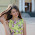## Pages

### C and corresponding Assembly (MSP430G2231)

Microcontroller programming become much simple if we use high level language like C. But it doesn't means that we could ignore the assembly language. In some situations, we might me forced to use a bit of assembly codes. Assembly language is a symbolic representation of a processor's native code. Using machine code allows the programmer to control precisely what the processor does. It offers a great deal of power to use all of the features of the processor. The resulting program is normally very fast and very compact. Using assembly programs, timings, for example, could be calculated very precisely.
Here, I am just posting some basic C codes and their corresponding assembly (generated by msp430-gcc). By comparing the compiler generated assembly and the source C file, I hope, we will get a good idea of how the compiler effectively utilize the stack, various addressing modes, functions, global variables, local variables, pointers etc etc.
For getting the disassembled view of the final hex code, we could use the msp430-objdump command. Disassembled view is the ideal one and it will contain each and every instruction from the reset address to the end of the program.
note:
[Today I found an interesting and simple msp430 assembler named naken430asm.

Below figure illustrates the 16 CPU registers and the memory organization. It is much important to know about the CPU registers and the memory organization before going to learn assembly language...

Example 1:

The launchpad have two leds connected to the P1.0 and P1.6. Those are helpful for testing simple programs...

Here,what I want to do is, just to turn on the led connected to the P1.0 (port 1 bit 0)
Below C program could be used for the purpose.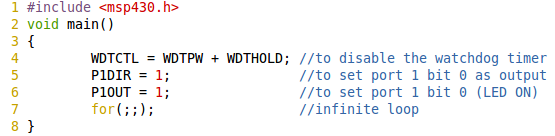The above file is saved as led.c

Now, using below command
msp430-gcc -mmcu=msp430g2231 -S led.c
an 'led.s' file (asm) is obtained. The main function in the led.s file is as below: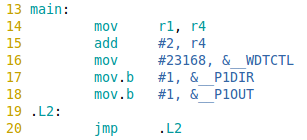A brief explanation to above code:
• Here , in line 14, the content in register r1(stack pointer) is moved to register r4. Initially the top of the stack ie 0x0280 is loaded (not shown in the above code) to r1 before the main function. r4 is generally used as frame pointer. In the current function main, if there are local variables, then the frame pointer will be used as reference for arranging the local variables in stack. But here there are no local variables used. But as a general procedure, compiler always uses the line.
• In next line 15, the frame pointer is set two bytes above the top of stack.
• In next line 16, number 23168 is moved to register WDTCTL.
• In line 17 and 18,  1 is moved to P1DIR and P1OUT (digital I/O registers)
• Now the line 19 and 20 constitutes an infinite loop.
Note: Using infinite loop is not a proper and ideal way to keep the processor static. This infinite loop still consumes power from the battery or equivalent source. There are some efficient power saving technique in the MSP430. This could be done by configuring the status register accordingly..

Example 2:

To toggle two LEDs (at P1.0 and P1.6)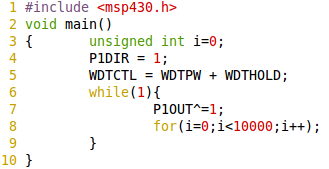assembly code: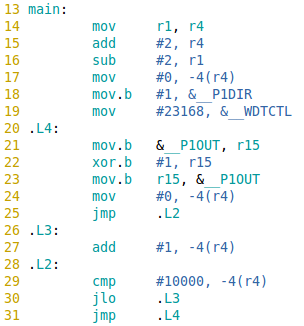Now, in the above assembly code , there is a local variable. So a space in stack is reserved for the local variable. The stack pointer is decreased by multiple of number of local variables.  In line number 17, wan see that the local variable 'i' is moved to stack by using the frame pointer as a reference. Now if more than one local variables are declared, then it will be stored in -6(r4), -8(r4) etc.. The while loop is maintained by the label '.L4' and the unconditional jump 'jmp .L4'. The for loop delay in the C (line 8) is maintained by the loop (from line 26 to 30)

When more local variables are there in the main, as below,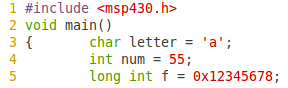the corresponding assembly is as below: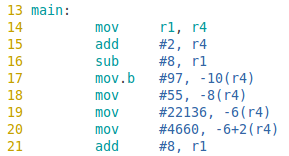Here, I used a three local variables ie char, int and long int . So a total of 8 bytes are to be reserved in stack. So in step 16, SP is decremented by 8.
Now, in case of long int 0x12345678, it requires 32 bits. This is done by splitting the 16 bit value to two 8 bit value and storing them in adjacent memory locations. (as in line 19 and 20)

Example 3: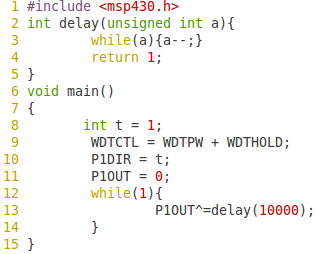assembly code: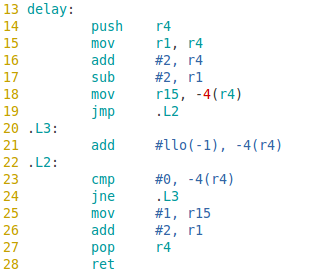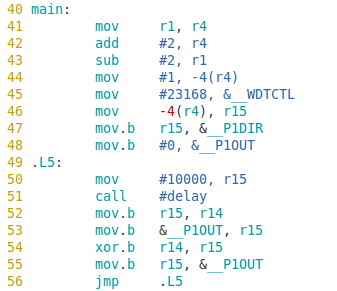In this case, delay function is called from main. The argument of the function is 10000 . This is introduced to the function via r15. Similarly, the return value from the delay function is obtained at the same argument register r15. The while loop (delay) is to obtain a small delay of about 1 second aprox so that we could observe the led blinking.
In case of a function call, what actually happening is, the assembler will convert the pseudo instruction 'call dst' to a combination of real instruction.

call subroutine:
1>address of the function is stored in a temp.
2>SP - 2 → SP
3>PC → @SP PC updated to TOS
4>tmp → PC dst saved to PC

Similarly, when returning from a function,  we use 'ret': But it is a pseudo instruction. Assembler will convert it to as below:

return subroutine:
1>@SP → PC
2>SP + 2 → SP
3>MOV @SP+,PC

Example 4:

(pointers)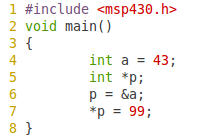The above code is a hello world example to illustrate pointers.
The corresponding assembly code is: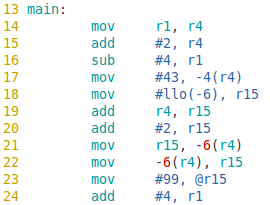A pointer hold an address. Pointer itself have a location in ram where the address of the pointed variable is stored. In above case, int a is defined and assigned value 43 to it. Now, the address of local variable 'a' is moved to p. For this, at first the address of the local variable a is obtained at r15. Then it is moved to the location of pointer ie -6(r4). Now, the pointer holds the address of local variable a.

1.2.